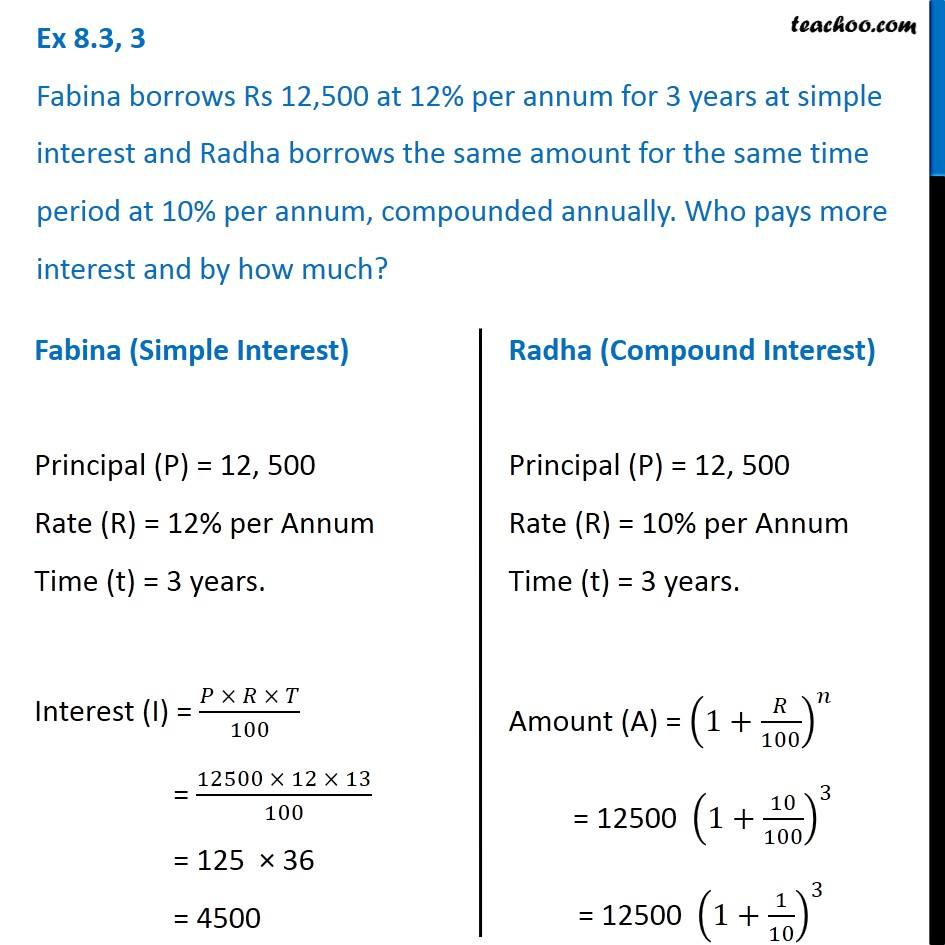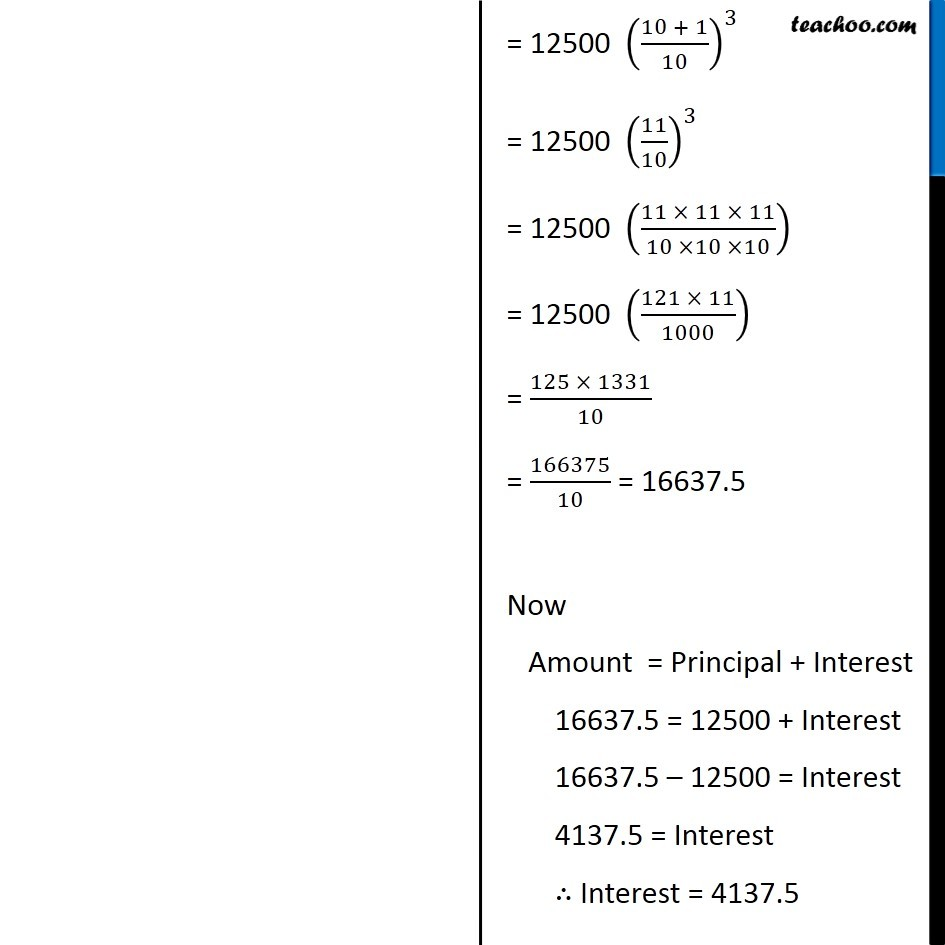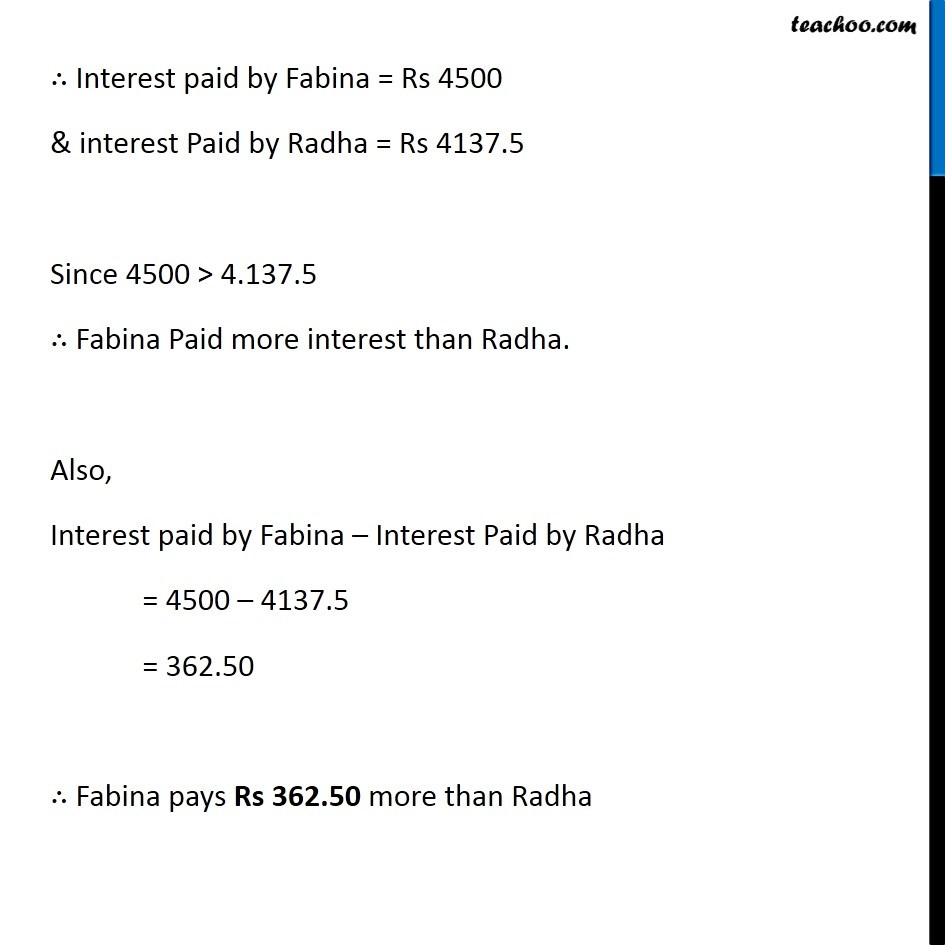Ex 7.3

Chapter 7 Class 8 Comparing Quantities
Serial order wiseLearn in your speed, with individual attention - Teachoo Maths 1-on-1 Class

### Transcript

Question 3 Fabina borrows Rs 12,500 at 12% per annum for 3 years at simple interest and Radha borrows the same amount for the same time period at 10% per annum, compounded annually. Who pays more interest and by how much? Fabina (Simple Interest) Principal (P) = 12, 500 Rate (R) = 12% per Annum Time (t) = 3 years. Interest (I) = (𝑃 × 𝑅 × 𝑇)/100 = (12500 × 12 × 13)/100 = 125 × 36 = 4500 Radha (Compound Interest) Principal (P) = 12, 500 Rate (R) = 10% per Annum Time (t) = 3 years. Amount (A) = (1+𝑅/100)^𝑛 = 12500 (1+10/100)^3 = 12500 (1+1/10)^3 = 12500 ((10 + 1)/10)^3 = 12500 (11/10)^3 = 12500 ((11 × 11 × 11)/(10 ×10 ×10)) = 12500 ((121 × 11)/1000) = (125 × 1331)/10 = 166375/10 = 16637.5 Now Amount = Principal + Interest 16637.5 = 12500 + Interest 16637.5 – 12500 = Interest 4137.5 = Interest ∴ Interest = 4137.5 ∴ Interest paid by Fabina = Rs 4500 & interest Paid by Radha = Rs 4137.5 Since 4500 > 4.137.5 ∴ Fabina Paid more interest than Radha. Also, Interest paid by Fabina – Interest Paid by Radha = 4500 – 4137.5 = 362.50 ∴ Fabina pays Rs 362.50 more than Radha